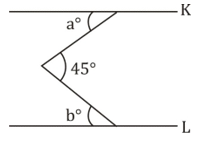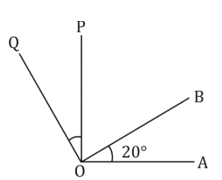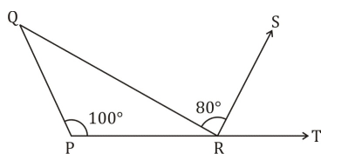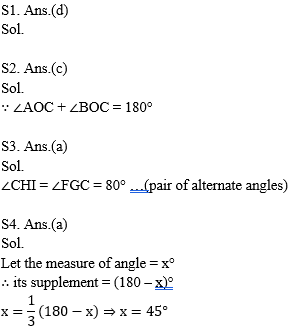Dear Students!!! There is most general as well as a scoring section in all the competitive entrance examinations in the teaching field i.e "Mathematics".Because in this section only one thing is work i.e your accuracy and that could be nourished with the daily practice. So, for this, we are providing you the daily quiz for all teaching exams i.e CTET Exam 2019DSSSB ,KVS,STET Exam.

Q1. Which of the following statements are true:
i. Angle forming a linear pair are supplementary.
ii. If two adjacent angles are equal, then measures of each angle will only be 90°.
iii. Angles forming a linear pair can both be acute angles.
iv. If angles forming a linear pair are equal, then each of these angles is of measure 90°.

(a) only i
(b) i, ii, iv
(c) iii and iv
(d) i and iv

Q2. AB is a straight line and O is a point on AB, if line OC is drawn not coinciding with OA or OB, then ∠AOC and ∠BOC are:

(a) equal
(b) complementary
(c) supplementary
(d) together equal to 100

Q3. In the given diagram AB∥ GH ∥DE and GF∥BD∥HI, ∠FGC = 80°. Find the value of ∠CHI:(a) 80°
(b) 120°
(c) 100°
(d) 160°

Q4. Angle is equal to one-third of its supplement. Find the measure:

(a) 45°
(b) 50°
(c) 55°
(d) None of these

Q5. In the figure below, lines K and L are parallel. The value of a° + b° is:(a) 45°
(b) 180°
(c) 160°
(d) 360°

Q6. In the figure, OP⊥OA and OQ⊥OB. Find ∠POQ if ∠AOB = 20°(a) 20°
(b) 30°
(c) 40°
(d) None of these

Q7. In the figure ∠PRQ = ∠SRT. If ∠QPR = 100° and ∠QRS = 80°, Find ∠PQR(a) 20°
(b) 30°
(c) 40°
(d) 60°(a) 220°
(b) 140°
(c) 150°
(d) None of these

Q9. In the given figure AB∥CD, ∠ABE = 100°, ∠MED = 25°. Find ∠CDE :(a) 125°
(b) 55°
(c) 65°
(d) 75°

Q10. A, O, B are three points on a line segment and C is a point not lying on AOB. If ∠AOC = 40° and OX, OY are the internal and external bisectors of ∠AOC and ∠BOC respectively, then ∠BOY is :

(a) 70°
(b) 80°
(c) 72°
(d) 68°

Solutions